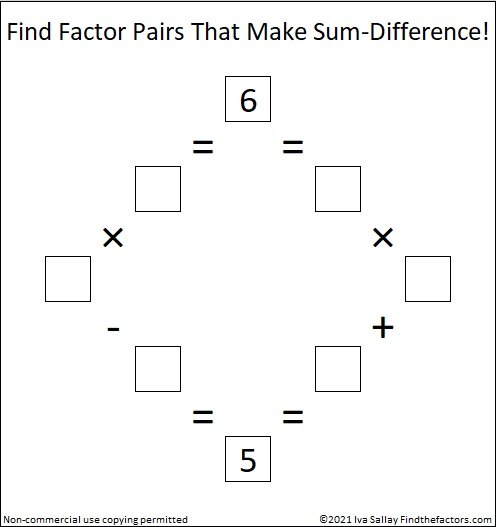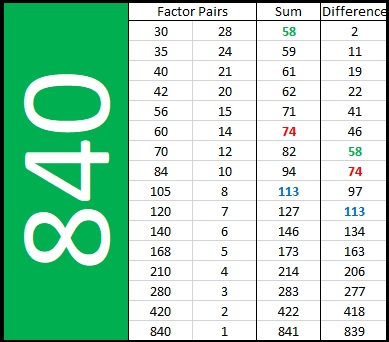# Sum-Difference Puzzles

Contents

### Ralph Waldo Emerson’s “Some Difference” Quote:

“The purpose of life is not to be happy. It is to be useful, to be honorable, to be compassionate, to have it make some difference that you have lived and lived well.” Ralph Waldo Emerson

### What Is a Sum-Difference Puzzle?

Sum-Difference Puzzles can be solved by elementary-aged children who have learned how to multiply and divide. For example, the factors of six and the number five have these relationships: 6 × 1 = 6 = 2 × 3, and 6 – 1 = 5 = 2 + 3. A Sum-Difference Puzzle asks that you put the factors, 6, 1, 2, and 3, in the appropriate boxes to make four true mathematical statements.What do the terms factor pair, sum, and difference mean?
A factor pair of a number is two whole numbers that result in that number when multiplied together. 2 × 3 and 6 × 1 show the factor pairs of 6.
A sum results from adding two or more numbers together. The sum of 2 and 3 is 5.
A difference results from subtracting two numbers. The difference of 6 and 1 is 5.

### Links to Posts That Contain a Sum-Difference Puzzle:

(Links in green include the primitive puzzle upon which it is based. Links in pink are primitive puzzles.)

### An Example of Factor Pairs’ Sums and Differences:

840 has 16 factor pairs. The sums of three of its factor pairs equal the differences of three other of its factor pairs.### An Application for Sum-Difference Puzzles:

The concept behind these puzzles will be useful in middle school when students need to recognize the difference in factors for similar-looking trinomials like x² + 5x – 6 and x² + 5x + 6.### What Numbers Can Be in a Sum-Difference Puzzle?

If a² + b² = c², and all three numbers are counting numbers, we say that a, b, and c form a Pythagorean triple. Furthermore, we can make a Sum-Difference puzzle based on those numbers: the top number in the Sum-Difference puzzle will be ab/2, and the bottom number will be c. The top number is always divisible by 6, and at least one of the prime factors of the bottom number will be one more than a multiple of four.

If the Pythagorean triple is a primitive, meaning the only common factor for a, b, and c is one, then the Sum-Difference puzzle will be a primitive as well.

If the Pythagorean triple can be expressed as ka, kb, kc where k is a whole number greater than 1, then the top number will be k²ab/2 and the bottom number will be kc. (And I will say that the Sum-Difference puzzle is really just a puzzle with the top number ab/2 and the bottom number c in disguise!)

### Sum-Difference Puzzles’ Relationship to Pythagorean Triples:

Here’s a specific example:
Suppose we want to use the quadratic formula to solve
2x² – 5x + 3 = 0 and 2x² – 5x – 3 = 0.
Let’s combine the left sides of those two equations into one expression:
2x² -5x ± 3.
The discriminant would be (-5)² – 4(2)(±3)
= 5² ± 4(2)(3)
= 5² ± 2(4)(3)
= 4² + 3² ± 2(4)(3), ( That’s because 4² + 3² = 5², since 3-4-5 is a Pythagorean triple.)
= 4² ± 2(4)(3) + 3²
= (4 ± 3)²
= 7² and 1², two odd perfect squares, each with an odd whole number square root!
And the complete quadratic formula will yield two integer number solutions for each equation, 2x² – 5x + 3 = 0 and 2x² – 5x – 3 = 0.